New update is available. Click here to update.

# Finding Power of Factorial Divisor

### Introduction:

We are given two numbers N and K; we need to find the largest power of K that divides N! (factorial of N). In other words, we need to find the power of the factorial divisor.

We should initially think about the instance of prime K. The unequivocal articulation for

N! = 1⋅ 2 ⋅ 3…( N − 1 ) ⋅ N

Note that each K-th component of the item is separable by K, for example, adds +1 to the appropriate response; the quantity of such elements is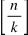Then, every K2-th component is detachable by K2, adding another +1 to the appropriate response (the main force of K, has effectively been included in the past section). The quantity of such components is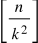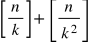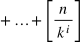The total is limited since just roughly the firstcomponents are not zeros. Hence, the runtime of this calculation is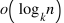Doesn't that sound confusing?

Let’s figure it out with the help of a hands-on example.

Let’s consider n = 5, k = 2. Value of 5! = 120 and the largest power of k that divides the factorial result i.e. largest power of 2 that divides 120 is 8 (23).

Source :Giphy

### Approach for Power of factorial in C++:

The efficient approach is based on Legendre’s formula. The following steps are followed: While dealing with this problem to find the maximum power of N that divides M factorial (M!), N can be anything prime or composite. Thus, in this case,

• First, the prime factors of a given number N are calculated.
• Then calculate the highest power from all prime factors that divides M!.
• Finally, we’ll calculate the prime factor with the lowest value of the highest power of N that divides M!.
• In other words, return the minimum of all calculated powers.As the minimum of both the numbers(35 and 70) is 35. So, the output is 35.

#### Output:

Note: if multiple powers of prime factors are present in n then divide the ‘count’ variable to get the maximum value of the factor.

#### Time Complexity:Here ‘N’ is the number for which we need to return the largest power of factorial of ‘fact’.

An additional loop is used to find the prime factors of number N. But we cannot directly multiply the complexity by sqrt(N) because ‘PowerPrime()’ is not directly dependent on sqrt(N) or N . ‘N’ will have the changing values with N = N / i and every time N changes, the value of sqrt(N) also changes.

#### Space Complexity: O(1)

The space complexity of this approach is O(1). As constant space is used.

What is a factorial?

The factorial of a number id defined by:

N! = N * (N - 1) * (N - 2) * … * 2 * 1

For example: 4! = 4 * 3 * 2 * 1 = 24.

How to find the divisors of a factorial?

Steps to find the divisors of a factorial:

• First, find the factorial of the given number.
• Then, count the total number of divisors of the factorial.

List the total divisors of 12!.

The divisors of 12 are 1, 2, 3, 4, 6, 12.

Is 18 a divisor of 6 and why?

No, 18 is not a divisor of 6. Because a divisor is one that divides opponent numbers either completely or with a remainder. And 18 don’t divide 6.

### Key Takeaways:

In this article, we learned how to find the power of factorial divisors, starting from brute force solution to an efficient approach, a discussion around its time complexity, and have gone through some hands-on examples. To get a grip on more such exciting problems, visit code studio

Happy coding